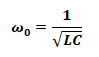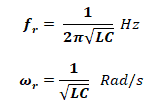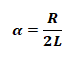# Equations & Formulas For RLC Circuits (Series & Parallel)

## RLC Circuits – Series & Parallel Equations & Formulas

RLC Circuit:

When a resistor, inductor and capacitor are connected together in parallel or series combination, it operates as an oscillator circuit (known as RLC Circuits) whose equations are given below in different scenarios as follow:### Parallel RLC Circuit

When they are connected in parallel combination

#### Impedance:

Total impedance of the circuit is;Where

• XL = Inductive reactance
• XC = Capacitive reactance

#### Power Factor:

The power factor for this circuit is

Cos θ = Z/R

#### Resonance Frequency:

When inductive reactance XL & capacitive reactance X­c of the circuit is equal.Where

• L = Inductance of inductor
• C = Capacitance of capacitor

#### Quality Factor:

It is the ratio of stored energy to the energy dissipated in the circuit.B.W = fr / Q

#### Resonant Circuit Current:

The total current through the circuit when the circuit is at resonance.

At resonance, the X= XC , so Z = R

IT = V/R

#### Current Magnification

Parallel resonance RLC circuit is also known current magnification circuit. Because, current flowing through the circuit is Q times the input current

Imag = Q IT

#### Characteristic Equation:#### Neper Frequency For Parallel RLC Circuit:#### Resonant Radian Frequency For Parallal RLC Circuit:#### Voltage Response:

• Over-Damped Response

When

ω0< α2

The roots s1 & s2 are real & distinct

• Under-Damped Response

When

ω0> α2

The roots s1 & s2 are complex & conjugate of each other

• Critically Damped Response

When

ω0= α2

The roots s1 & s2 are real & equal.

### Series RLC Circuit:

#### Impedance:

The total impedance of the series RLC circuit is;#### Power Factor:

The power factor of Series RLC circuit;

Cos θ = R/Z

#### Resonance Frequency:

The frequency at which the inductive reactance XL = Capacitive reactance X­­c is known as resonance frequency.Where

• L = Inductance of the inductor
• C = Capacitance of the capacitor

#### Quality Factor:#### Bandwidth:

B.W = (fr / Q)

• B.W = (R / L)              in rad/s
• B.W = (R / 2πL)         in hz

fh = fr + ½ B.W

fl = fr – ½ B.W

#### Characteristic Equation:#### Neper Frequency For series RLC Circuit:#### Resonant Radian Frequency For series RLC Circuit:#### Voltage Response:

• Over-Damped Response

When

ω0< α2

The roots s1 & s2 are real & distinct

• Under-Damped Response

When

ω0> α2

The roots s1 & s2 are complex & conjugate of each other

• Critically Damped Response

When

ω0= α2

The roots s1 and s2 are real & equal

Related Formulas and Equations Posts: